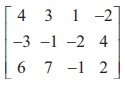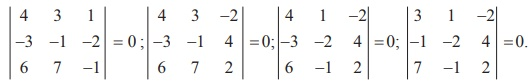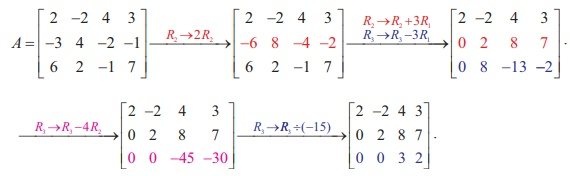Home | | Maths 12th Std | Rank of a Matrix

# Rank of a Matrix

To define the rank of a matrix, we have to know about sub-matrices and minors of a matrix.

Rank of a Matrix

To define the rank of a matrix, we have to know about sub-matrices and minors of a matrix.

Let A be a given matrix. A matrix obtained by deleting some rows and some columns of A is called a sub-matrix of A. A matrix is a sub-matrix of itself because it is obtained by leaving zero number of rows and zero number of columns.

Recall that the determinant of a square sub-matrix of a matrix is called a minor of the matrix.

### Definition 1.6

The rank of a matrix A is defined as the order of a highest order non-vanishing minor of the matrix A. It is denoted by the symbol Žü (A). The rank of a zero matrix is defined to be 0.

### Note

i.                     If a matrix contains at-least one non-zero element, then Žü ( A) Ōēź 1.

ii.                     The rank of the identity matrix In is n.

iii.                     If the rank of a matrix A is r, then there exists at-least one minor of A of order r which does not vanish and every minor of A of order r +1 and higher order (if any) vanishes.

iv.                     If A is an m ├Ś n matrix, then Žü (A) Ōēż min{m, n} = minimum of m, n.

v.                     A square matrix A of order n has inverse if and only if Žü ( A) = n.

### Example 1.15

Find the rank of each of the following matrices:Solution

(i) Let A =. Then A is a matrix of order 3├Ś 3. So Žü(A) Ōēż min {3, 3}  = 3. The highest  order of minors of A is 3 . There is only one third order minor of A .

It is=  3 (6ŌłÆ 6) ŌłÆ 2 (6ŌłÆ6) +  5 (3 ŌłÆ 3) = 0. So, Žü(A) < 3.

Next consider the second-order minors of A .

We find that the second order minor= 3 ŌłÆ 2 = 1 ŌēĀ 0 . So Žü(A) = 2 .

(ii) Let A =. Then A is a matrix of order 3├Ś4 . So Žü(A) Ōēż min {3, 4}  = 3.

The highest order of minors of A is 3 . We search for a non-zero third-order minor of A . But

we find that all of them vanish. In fact, we haveSo, Žü(A) < 3. Next, we search for a non-zero second-order minor of A .

We find that= -4+9 =5 ŌēĀ 0 . So, Žü(A) = 2 .

### Remark

Finding the rank of a matrix by searching a highest order non-vanishing minor is quite tedious when the order of the matrix is quite large. There is another easy method for finding the rank of a matrix even if the order of the matrix is quite high. This method is by computing the rank of an equivalent row-echelon form of the matrix. If a matrix is in row-echelon form, then all entries below the leading diagonal (it is the line joining the positions of the diagonal elements a11 , a22 , a33 ,L. of the matrix) are zeros. So, checking whether a minor is zero or not, is quite simple.

### Example 1.16

Find the rank of the following matrices which are in row-echelon form :Solution

(i) Let A =. Then A is a matrix of order 3 3 ├Ś and Žü(A) Ōēż 3

The third order minor |A| == (2) (3)( 1) = 6 ŌēĀ 0 . So, Žü(A) = 3 .

Note that there are three non-zero rows.

(ii) Let A =. Then A is a matrix of order 3├Ś 3 and Žü(A) Ōēż 3.

The only third order minor is |A| == (-2) (5) (0) = 0 . So Žü(A) Ōēż 2 .

There are several second order minors. We find that there is a second order minor, for  example,= (-2)(5) = -10 ŌēĀ 0 . So, Žü(A) = 2.

Note that there are two non-zero rows. The third row is a zero row.

(iii) Let A =. Then A is a matrix of order 4 ├Ś 3 and Žü(A) Ōēż 3.

The last two rows are zero rows. There are several second order minors. We find that there  is a second order minor, for example,= (6) (2) = 12 ŌēĀ 0 . So, Žü(A) = 2.

Note that there are two non-zero rows. The third and fourth rows are zero rows.

We observe from the above example that the rank of a matrix in row echelon form is equal  to the number of non-zero rows in it. We state this observation as a theorem without proof.

Theorem 1.11

The rank of a matrix in row echelon form is the number of non-zero rows in it.

The rank of a matrix which is not in a row-echelon form, can be found by applying the following result which is stated without proof.

Theorem 1.12

The rank of a non-zero matrix is equal to the number of non-zero rows in a row-echelon form of the matrix.

### Example 1.17

Find the rank of the matrixby reducing it to a row-echelon form.

### Solution

Let A =. Applying elementary row operations, we getThe last equivalent matrix is in row-echelon form. It has two non-zero rows. So, Žü (A)= 2.

### Example 1.18

Find the rank of the matrixby reducing it to a row-echelon form.

### Solution

Let A be the matrix. Performing elementary row operations, we getThe last equivalent matrix is in row-echelon form. It has three non-zero rows. So, Žü(A) = 3 .

Elementary row operations on a matrix can be performed by pre-multiplying the given matrix by a special class of matrices called elementary matrices.

### Definition 1.7

An elementary matrix is defined as a matrix which is obtained from an identity matrix by applying only one elementary transformation.

### Remark

If we are dealing with matrices with three rows, then all elementary matrices are square matrices of order 3 which are obtained by carrying out only one elementary row operations on the unit matrix I3. Every elementary row operation that is carried out on a given matrix A can be obtained by pre-multiplying A with elementary matrix. Similarly, every elementary column operation that is carried out on a given matrix A can be obtained by post-multiplying Awith an elementary matrix. In the present chapter, we use elementary row operations only.

For instance, let us consider the matrix A =Suppose that we do the transformation R2  ŌåÆ R2 + ╬╗R3 on A, where ╬╗ ŌēĀ 0 is a constant. Then, we getThe matrixis an elementary matrix, since we havePre-multiplying A by, we getFrom (1) and (2), we getSo, the effect of applying the elementary transformation R2 ŌåÆ R2 + ╬╗R3 on A is the same as that of pre-multiplying the matrix A with the elementary matrixSimilarly, we can show that

(i) the effect of applying the elementary transformation R2Ōåö R3 on A is the same as that of  pre-multiplying the matrix A with the elementary matrix(ii) the effect of applying the elementary transformation R2 ŌåÆ R2╬╗ on A is the same as that of  pre-multiplying the matrix A with the elementary matrixWe state the following result without proof.

### Theorem 1.13

Every non-singular matrix can be transformed to an identity matrix, by a sequence of elementary row operations.

As an illustration of the above theorem, let us consider the matrix AThen, |A| =  12+ 3 = 15 ŌēĀ  0. So, A is non-singular. Let us transform A into I2 by a sequence of  elementary row operations. First, we search for a row operation to make a11 of A as 1. The elementary row operation needed for this is R1 ŌåÆ (1/2) R1. The corresponding elementary matrix isNext, let us make all elements below a11 of E1A as 0. There is only one element a21.

The elementary row operation needed for this is R2  ŌåÆ R2  + (ŌłÆ3) R1 .

The corresponding elementary matrix is E2 =Next, let us make a22 of E2(E2A) as 1. The elementary row operation needed for this isThe corresponding elementary matrix is E3Then, we get E3(E2(E1A)) =Finally, let us find an elementary row operation to make a12 of E3(E2(E1A)) as 0. The elementary  row operation needed for this is R1 ŌåÆ R1 + (1/2) R2. The corresponding elementary matrix isWe write the above sequence of elementary transformations in the following manner:### Example 1.19

Show that the matrixis non-singular and reduce it to the identity matrix by elementary row transformations.

### Solution

Let A =.Then, |A| = 3 (0+2 ) ŌĆō 1(2+5) + 4(4-0) = 6-7+16 ŌēĀ 0. So, A is non-singular. Keeping the identity matrix as our goal, we perform the row operations sequentially on A as follows:Tags : Definition, Theorem, Formulas, Solved Example Problems | Elementary Transformations of a Matrix , 12th Mathematics : UNIT 1 : Applications of Matrices and Determinants
Study Material, Lecturing Notes, Assignment, Reference, Wiki description explanation, brief detail
12th Mathematics : UNIT 1 : Applications of Matrices and Determinants : Rank of a Matrix | Definition, Theorem, Formulas, Solved Example Problems | Elementary Transformations of a Matrix